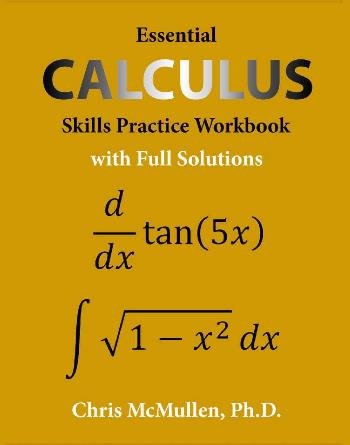Šta je novo?

# Essential Calculus Skills Practice Workbook with Full Solutions

• Angelus#### AngelusEssential Calculus Skills Practice Workbook with Full Solutions
by Chris McMullen
Zishka Publishing | August 2018 | ISBN-10: 1941691242 | ePUB | 24.2 mb

https://www.amazon.com/Essential-Calculus-Practice-Workbook-Solutions/dp/1941691242

The author, Chris McMullen, Ph.D., has over twenty years of experience teaching math skills to physics students. He prepared this comprehensive workbook (with full solutions to every problem) to share his strategies for mastering calculus. This workbook covers a variety of essential calculus skills, including:

- derivatives of polynomials, trig functions, exponentials, and logarithms
- the chain rule, product rule, and quotient rule
- second derivatives
- how to find the extreme values of a function
- limits, including l’Hopital’s rule
- antiderivatives of polynomials, trig functions, exponentials, and logarithms
- definite and indefinite integrals
- techniques of integration, including substitution, trig sub, and integration by parts
- multiple integrals

The goal of this workbook isn’t to cover every possible topic from calculus, but to focus on the most essential skills needed to apply calculus to other subjects, such as physics or engineering.

CONTENTS
Introduction
1 DERIVATIVES OF POLYNOMIALS
2 THE CHAIN RULE, PRODUCT RULE, AND QUOTIENT RULE
3 DERIVATIVES OF TRIG FUNCTIONS
4 DERIVATIVES OF EXPONENTIALS
5 DERIVATIVES OF LOGARITHMS
6 SECOND DERIVATIVES
7 EXTREME VALUES
8 LIMITS AND L’HÔPITAL’S RULE
9 INTEGRALS OF POLYNOMIALS
10 DEFINITE INTEGRALS
11 INTEGRALS OF TRIG FUNCTIONS
12 INTEGRALS OF EXPONENTIALS AND LOGARITHMS
13 INTEGRATION BY POLYNOMIAL SUBSTITUTION
14 INTEGRATION BY TRIGONOMETRIC SUBSTITUTION
15 INTEGRATION BY PARTS
16 MULTIPLE INTEGRALS

TORRENT
Hidden content
You need to react to this post in order to see this content.
OR

MAGNET
Hidden content
You need to react to this post in order to see this content.